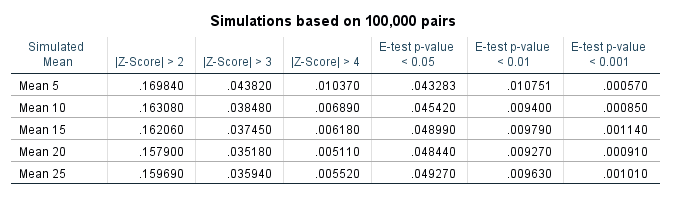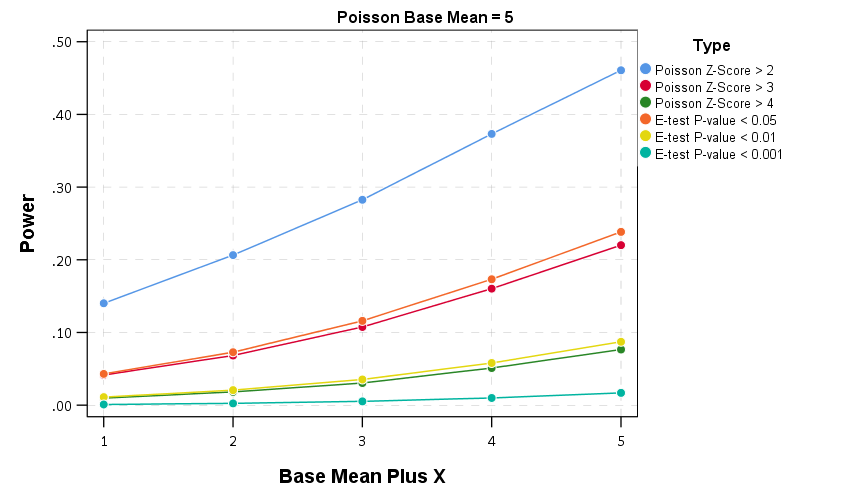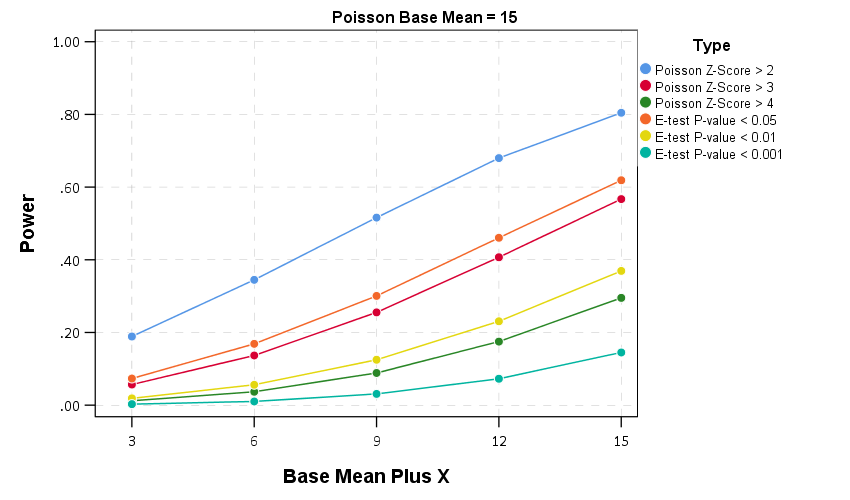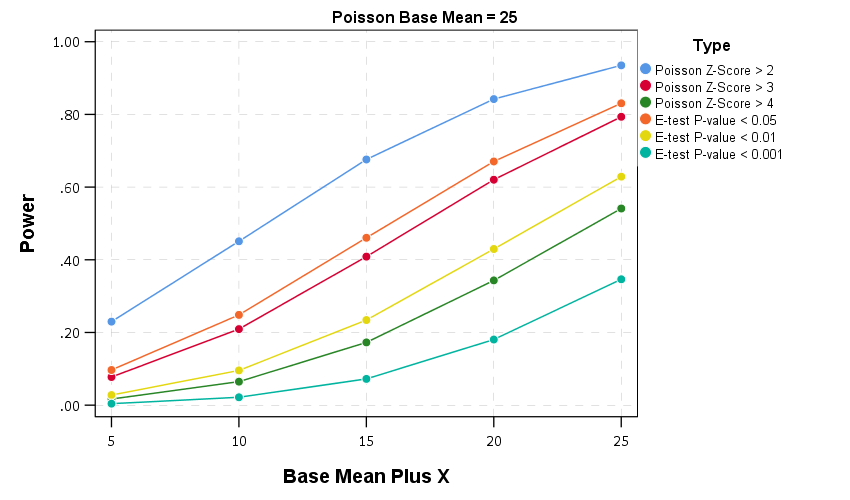# Testing changes in short run crime patterns: The Poisson e-test

A common task for a crime analyst is to see if a current set of crime numbers is significantly rising. For a typical example, in prior data there are on average 16 robberies per month, so are the 25 robberies that occurred this month a significant change from the historical pattern? Before I go any further:

PERCENT CHANGE IS A HORRIBLE METRIC — PLEASE DO NOT USE PERCENT CHANGE ANYMORE

But I cannot just say don’t use X — I need to offer alternatives. The simplest is to just report the change in the absolute number of crimes and let people judge for themselves whether they think the increase is noteworthy. So you could say in my hypothetical it is an increase of 9 crimes. Not good, but not the end of the world. See also Jerry Ratcliffe’s different take but same general conclusion about year-to-date percent change numbers.

Where this fails for the crime analyst is that you are looking at so many numbers all the time, it is difficult to know where to draw the line to dig deeper into any particular pattern. Time is zero-sum, if you spend time looking into the increase in robberies, you are subtracting time from some other task. If you set your thresholds for when to look into a particular increase too low, you will spend all of your time chasing noise — looking into crime increases that have no underlying cause, but are simply just due to the random happenstance. Hence the need to create some rules about when to look into crime increases that can be applied to many different situations.

For this I have previously written about a Poisson Z-score test to replace percent change. So in our original example, it is a 56% increase in crimes, `(25-16)/16 = 0.5625`. Which seems massive when you put it on a percent change scale, but only amounts to 9 extra crimes. But using my Poisson Z-test, which is simply `2 * [ Square_Root(Current) - Square_Root(Historical) ]` and follows an approximate standard normal distribution, you end up with:

`2*(sqrt(25) - sqrt(16)) = 2*(5 - 4) = 2`

Hearkening back to your original stats class days, you might remember a z-score of plus or minus 2 has about a 0.05 chance in occurring (1 in 20). Since all analysts are monitoring multiple crime patterns over time, I suggest to up-the-ante beyond the usual plus or minus 2 to the more strict plus or minus 3 to sound the alarm, which is closer to a chance occurrence of 1 in 1000. So in this hypothetical case there is weak evidence of a significant increase in robberies.

The other day on the IACA list-serve Isaac Van Patten suggested to use the Poisson C-test via this Evan Miller app. There is actually a better test than that C-test approach, see A more powerful test for comparing two Poisson means, by Ksrishnamoorthy and Thomson (2004), which those authors name as the E-test (PDF link here). So I just examine the E-test here and don’t worry about the C-test.

Although I had wrote code in Python and R to conduct the e-test, I have never really studied it. In this example the e-test would result in a p-value rounded to 0.165, so again not much evidence that the underlying rate of changes in the hypothetical example.

My Poisson Z-score wins in terms of being simple and easy to implement in a spreadsheet, but the Poisson e-test certainly deserves to be studied in reference to my Poisson Z-score. So here I will test the Poisson e-test versus my Poisson Z-score approach using some simulations. To do this I do two different tests. First, I do a test where the underlying Poisson distribution from time period to time period does not change at all, so we can estimate the false positive rate for each technique. The second I introduce actual changes into the underlying crime patterns, so we can see if the test is sensitive enough to actually identify when changes do occur in the underlying crime rate. SPSS and Python code to replicate this simulation can be downloaded from here.

# No Changes and the False Positive Rate

First for the set up, I generate 100,000 pairs of random Poisson distributed numbers. I generate the Poisson means to have values of 5, 10, 15, 20 and 25. Since each of these pairs is always the same, any statistically significant differences are just noise chasing. (I limit to a mean of 25 as the e-test takes a bit longer for higher integers, which is not a big deal for an analyst in practice, but is for a large simulation!)

Based on those simulations, here is a table of the false positive rate given both procedures and different thresholds.1So you can see my Poisson Z-score has near constant false positive rate for each of the different means, but the overall rate is higher than you would expect from the theoretical standard normal distribution. My advice to up the threshold to 3 only limits the false positive rate for this data to around 4 in 100, whereas setting the threshold to a Z-score of 4 makes it fewer than 1 in 100. Note these are false positives in either direction, so the false positive rate includes both false alarms for significantly increasing trends as well as significantly decreasing trends.

The e-test is as advertised though, the false positive rate is pretty much exactly as it should be for p-values of less than 0.05, 0.01, and 0.001. So in this round the e-test is a clear winner based on false positives over my Poisson Z-score.

# Testing the power of each procedure

To be able to test the power of the procedure, I add in actual differences to the underlying Poisson distributed random values and then see if the procedure identifies those changes. The differences I test are:

• base 5, add in increase of 1 to 5 by 1
• base 15, add in increase of 3 to 15 by 3
• base 25, add in increase of 5 to 25 by 5

I do each of these for pairs of again 100,000 random Poisson draws, then see how often the procedure flags the the second value as being significantly larger than the first (so I don’t count bad inferences in the wrong direction). Unlike the prior simulation, these numbers are always different, so a test with 100% power would always say these simulated values are different. No test will ever reach that level of power though for tiny differences in Poisson data, so we see what proportion of the tests are flagged as different, and that proportion is the power of the test. In the case with tiny changes in the underlying Poisson distribution, any test will have less power, so you evaluate the power of the test over varying ranges of actual differences in the underlying data.

Then we can draw the power curves for each procedure, where the X axis is the difference from the underlying Poisson distribution, and the Y axis is the proportion of true positives flagged for each procedure.2 A typical "good" amount of power is considered to be 0.80, but that is more based on being a simple benchmark to aim for in experimental designs than any rigorous reasoning that I am aware of.So you can see there is a steep trade-off in power with setting a higher threshold for either the Poisson Z score or the E-test. The curves for the Z score of above 3 and above 4 basically follow the E-test curves for <0.05 and <0.01. The Poisson Z-score of over 2 has a much higher power, but of course that comes with the much higher false positive rate as well.

For the lowest base mean of 5, even doubling the underlying rate to 10 still has quite low power to uncover the difference via any of these tests. With bases of 15 and 25 doubling gets into a bit better range of at least 0.5 power or better. Despite the low power though, the way these statistics are typically implemented in crime analysis departments along regular intervals, I think doing a Poisson Z-score of > 3 should be the lowest evidentiary threshold an analyst should use to say "lets look into this increase further".

Of course since the E-test is better behaved than my Poisson Z-score you could swap that out as well. It is a bit harder to implement as a simple spreadsheet formula, but for those who do not use R or Python I have provided an excel spreadsheet to test the differences in two simple pre-post counts in the data files to replicate this analysis.

# In conclusion

I see a few things to improve upon this work in the future.

First is that given the low power, I wonder if there is a better way to identify changes when monitoring many series but still be able to control the false positive rate. Perhaps some lower threshold for the E-test but simultaneously doing a false discovery rate correction to the p-values, or maybe some way to conduct partial pooling of the series into a multi-level model with shrinkage and actual parameters of the increase over time.

A second is a change in the overall approach about how such series are monitored, in particular using control charting approaches in place of just testing one vs another, but to identify consistent rises and falls. Control charting is tricky with crime data — there is no gold standard for when an alarm should be sounded, crime data show seasonality that needs to be adjusted, and it is unclear when to reset the CUSUM chart — but I think those are not unsolvable problems.

One final thing I need to address with future work is the fact that crime data is often over-dispersed. For my Poisson Z-score just setting the threshold higher with data seemed to work ok for real and simulated data distributed like a negative binomial distribution, but I would need to check whether that is applicable to the e-test as well. I need to do more general analysis to see the typical amounts of over/under dispersion though in crime data to be able to generate a reasonable simulation though. I can probably use NIBRS data to figure that out — so for the next blog post!

1. Note the e-test is not defined when both values are zero.

2. You can technically calculate the exact power of the e-test, see the cited Ksrishnamoorthy & Thomson (2004) article that introduces it. For simplicity I am just doing the simulation for both my Poisson Z-scores and the e-test here.

1.#### T.L. Brink

/  June 15, 2021

This is fascinating. I have long suspected that the Poisson was a better representation of many real-world phenomena (compared to Gauss). Are you aware of any online e-test calculators (or could you create one).

•#### apwheele

/  June 15, 2021

While some online apps are on my bucketlist to figure out, I would not hold my breath. It is easiest in R to replicate. If you do this:

#######
source(‘https://dl.dropboxusercontent.com/s/r4zmlxmi41tqsts/ETest.R?dl=0’)
poisson.etest(5,3)
#######

You can just do the etest on your own data with two lines.